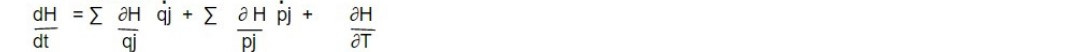# Physical Significance of Hamiltonian

The Hamiltonian in classical and quantum mechanics is a mathematical object that encodes the total energy of a physical system. It plays a central role in determining the time evolution of a system and is used to formulate the laws of motion in terms of equations of motion for the system's variables. The Hamiltonian is therefore a key quantity in understanding the dynamics and behaviour of physical systems, making it of great physical significance.

ELI5: Imagine the Hamiltonian is like a pot of energy for a system, like a toy car or a ball. It tells us how much energy the system has and how it is being used. By knowing the Hamiltonian, we can understand how the system will move and change over time, just like how you can know how your toy car will move when you push it, or how your ball will roll. The Hamiltonian helps us explain why things happen in the way they do.

Like Lagrangian, H also posses the dimensions of energy but in all circumstances it is not equal to the total energy E. Restriction for this equality, i.e., E= H are:

1. The system be conservative one, i.e. P.E is coordinate dependent and not velocity dependent and

2. Coordinate transformation equations be independent of time, so that `∑pjqj = 2T`

There conditions are necessary for Hamiltonian H to represent the total energy E. It may happen that though coordinate transformation equation involve time explicitly yet L be still independent of time, then

`H = ∑pjq̇j - L(qj, q̇j)` will be a constant i.e H will continue to be a constant of motion but not total energy. It can be shown that it does not occur in Lagrangian L then Hamiltonian H will not involve t. Since

H = H(qj,pj,t)Also from canonical equation,

∂H/ ∂qj = -ṗj and ∂H/ ∂pj = q̇j

Therefore dH/dt = - ∑ṗjq̇j + ∑ṗjq̇j + ∂H/ ∂t

But ∂H/ ∂t = - ∂L/ ∂t

If L does not involve time t, then ∂L/ ∂t =0 shows that

∂H/ ∂t = 0

So `dH/dt =0`

Which mean t will not appear in H. Cyclic Hamiltonian is constant of motion.

This note is taken from Classical Mechanics, Msc physics, Nepal.

This note is a part of the Physics Repository.